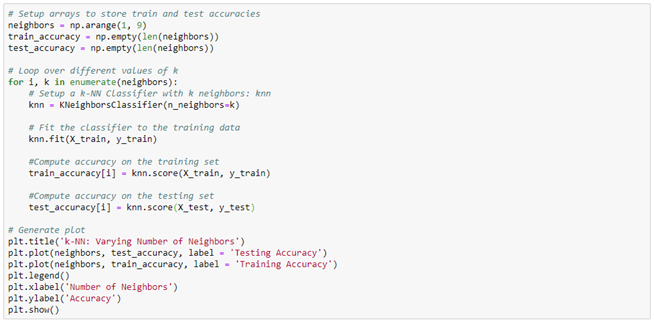Search

# Statistical Data Analytics In Machine Learning

In this blog you learn some important machine learning algorithms using MNIST Dataset:

### Table of content

1. k-Nearest Neighbors.

2. Linear Regression.

3. Support Vector Machines.

4. Naïve Bayes.

5. Model Evaluation.

6. Exercises.

In this exercise, you'll be working with the MNIST digits recognition dataset, which has 10 classes, the digits 0 through 9! A reduced version of the MNIST dataset is one of scikit-learn's included datasets, and that is the one we will use in this exercise.

Each sample in this scikit-learn dataset is an 8x8 image representing a handwritten digit. Each pixel is represented by an integer in the range 0 to 16, indicating varying levels of black.

To load dataset, using the following code:Display a random number to verify the datasetOutput:Before applying the classifier, we need to split the dataset into training and testing parts.### 1. k-Nearest Neighbors

Build KNN classifier for the above dataset1.1 Varying Number of Neighbours

In this exercise, you need to compute and plot the training and testing accuracy scores with different values of k (e.g. 1 to 8).Output:1.2 Overfitting vs. Underfitting

Which values of k makes the discrepancy between training accuracy and testing accuracy bigger or smaller? Which case is underfitting and which case is overfitting? Explain why.

### 2. Linear Regression

Build Linear Regression classifier using the same dataset### 3. Support Vector Machines

In this section, you need to compute the accuracy scores of the same dataset using SVM classifiers.### 4. Naïve Bayes

Classify above dataset using Naïve Bayes classifier.### 5. Model Evaluation

Compare the accuracy of different classifiers in the plot.Output:### Practice Exercises

In this part, you will be working with the Iris dataset

• Load this dataset from scikit-learn

• Classify using following techniques (kNN, Bayes, SVM).

• Compare the accuracy of different classifiers in the plot